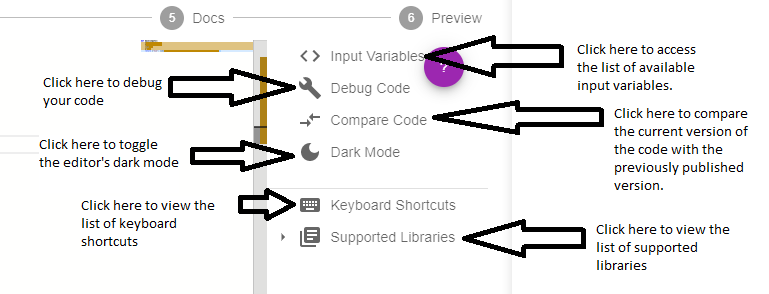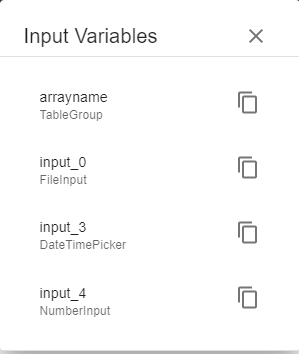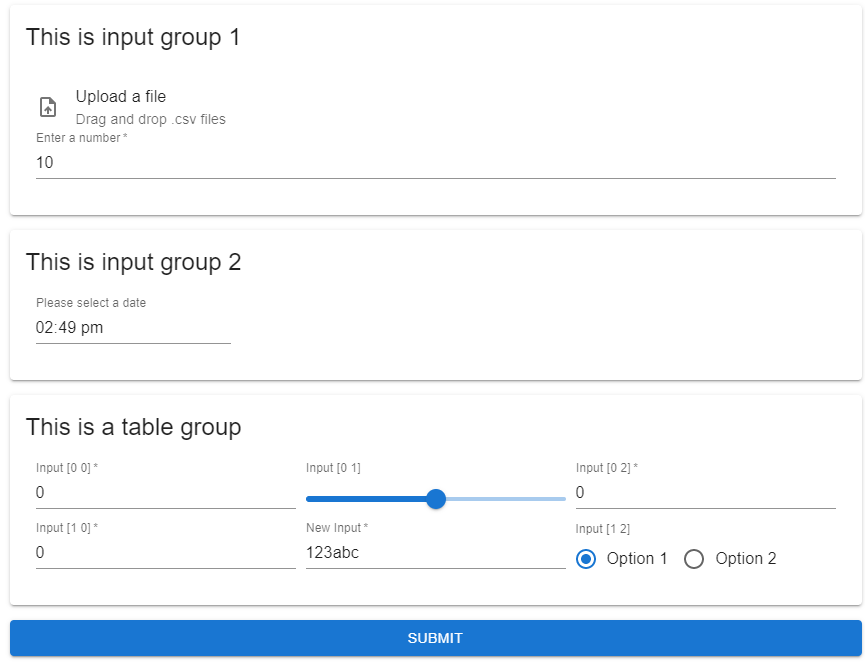# Overview

The Code step is responsible for running calculations on the user inputs with Python code, and then returning the computed values or visual diagrams to be displayed in the outputs. In this step, the developer can write, debug, and compare Python code.

The def main(inputs): function is required, and makes the connection between inputs, code, and outputs. The inputs of the main function is a dictionary whose keys are the unique variable names defined in the Inputs step, and the values are the user inputs. The main function is expected to return a dictionary whose key-value pairs are to be used in the output step.

This step has a smart code editor (similar to VSCode) equipped with syntax highlighting, debugging, keyboard shortcuts, and code comparison. In addition, the input variables defined in the Inputs step can be accessed by clicking on <> Input Variables. The image below provides a description the code editor toolbar:As an example, the function main(inputs) below provides the sum and product of two numerical input variables var 1 and var 2 and returns a dictionary of four keys: "Sum", "Product", "First Variable", and "Second Variable".

def main(inputs):    a = inputs['var_1']    b = inputs['var_2']    suma = a+b    pr = a*b    return {"Sum": suma, "Product":pr, "First Variable":a, "Second Variable":b}

## Input Variables​

Clicking on <> Input Variables provides the developer with a list of input variables that can be copied and pasted into the code editor. The pasted code will be a variable that contains the user inputted value.

As an example, the image below shows the four input variables arrayname, input_0, input_3, and input_4, where FileInput, DateTimePicker, and NumberInput are input types, and arrayname is a nested array of values from the Table Group.These input variables correspond to the following inputs page:## Supported Libraries​

The list of supported Python libraries can be shown by clicking on Supported Libraries in the toolbar. In order to use any of the supported libraries, the developer must first import the library as traditionally done in Python programs.

For example, the following code imports the numpy library and uses one of the associated functions on a numerical input.

import numpy as npdef main(inputs):    a = inputs['var_1']    l = np.log(a)    return{"Logarithm",l}
tip

If your desired Python library is not supported, then add a feature request here. If there is enough users wanting to use it, then it will be installed.